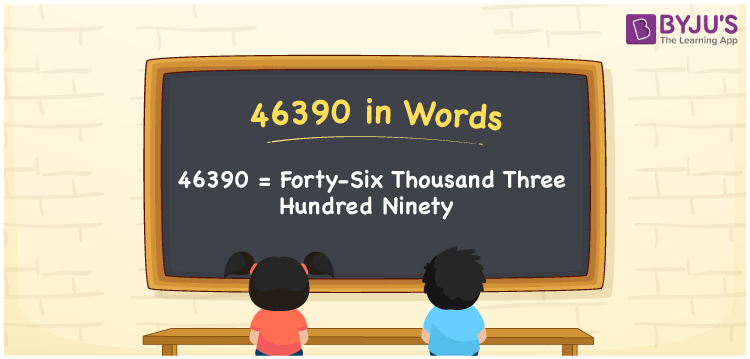# 46390 in Words

We can write 46390 in words as Forty-six thousand three hundred ninety. If you are spending Rs. 46390 for bike maintenance in one year, then you can say, “Am spending Forty-six thousand three hundred ninety rupees for bike maintenance in one year”. In this article, you will understand how to convert the cardinal number 46390 into words.

 46390 in words Forty-six thousand three hundred ninety Forty-six thousand three hundred ninety in Numbers 46390

## 46390 in English words

Generally, we write numbers in words using the English alphabet. So, we spell 46390 in English as “Forty-six thousand three hundred ninety”.## How to Write 46390 in Words?

To learn how to write the number 46390 in words, we need to make a place value chart with five columns since it is a five-digit number.

 Ten thousand Thousands Hundreds Tens Ones 4 6 3 9 0

Here, ones = 0, tens = 9, hundreds = 3, thousands = 6, ten thousands = 4

Thus, 4 × Ten thousand + 6 × Thousand + 3 × Hundred + 9 × Ten + 0 × One

= 4 × 10000 + 6 × 1000 + 3 × 100 + 9 × 10 + 0 × 1

= 40000 + 6000 + 300 + 90

= Forty thousand + Six thousand + Three hundred + Ninety

= Forty-six thousand three hundred ninety

Therefore, 46390 in words = Forty-six thousand three hundred ninety.

### Facts About the Number 46390

As we know, 46390 is a natural number that is the successor of 46389 and the predecessor of 46391.

46390 in words – Forty-six thousand three hundred ninety

Is 46390 an odd number? – No

Is 46390 an even number? – Yes

Is 46390 a perfect square number? – No

Is 46390 a perfect cube number? – No

Is 46390 a prime number? – No

Is 46390 a composite number? – Yes

## Frequently Asked Questions on 46390 in Words

Q1

### How do you write 46390 in English words?

The number 46390 can be written in English words as Forty-six thousand three hundred ninety.
Q2

### How to write Rs. 46390 in words on a cheque?

On a cheque, we generally write Rs. 46390 in words as “Forty-six thousand three hundred ninety rupees only”.
Q3

### Find 46390 + 10610. Express answer in words

46390 + 10610 = 57000 Thus, the value of 46390 + 10610, i.e. 57000 in words is Fifty-seven thousand.